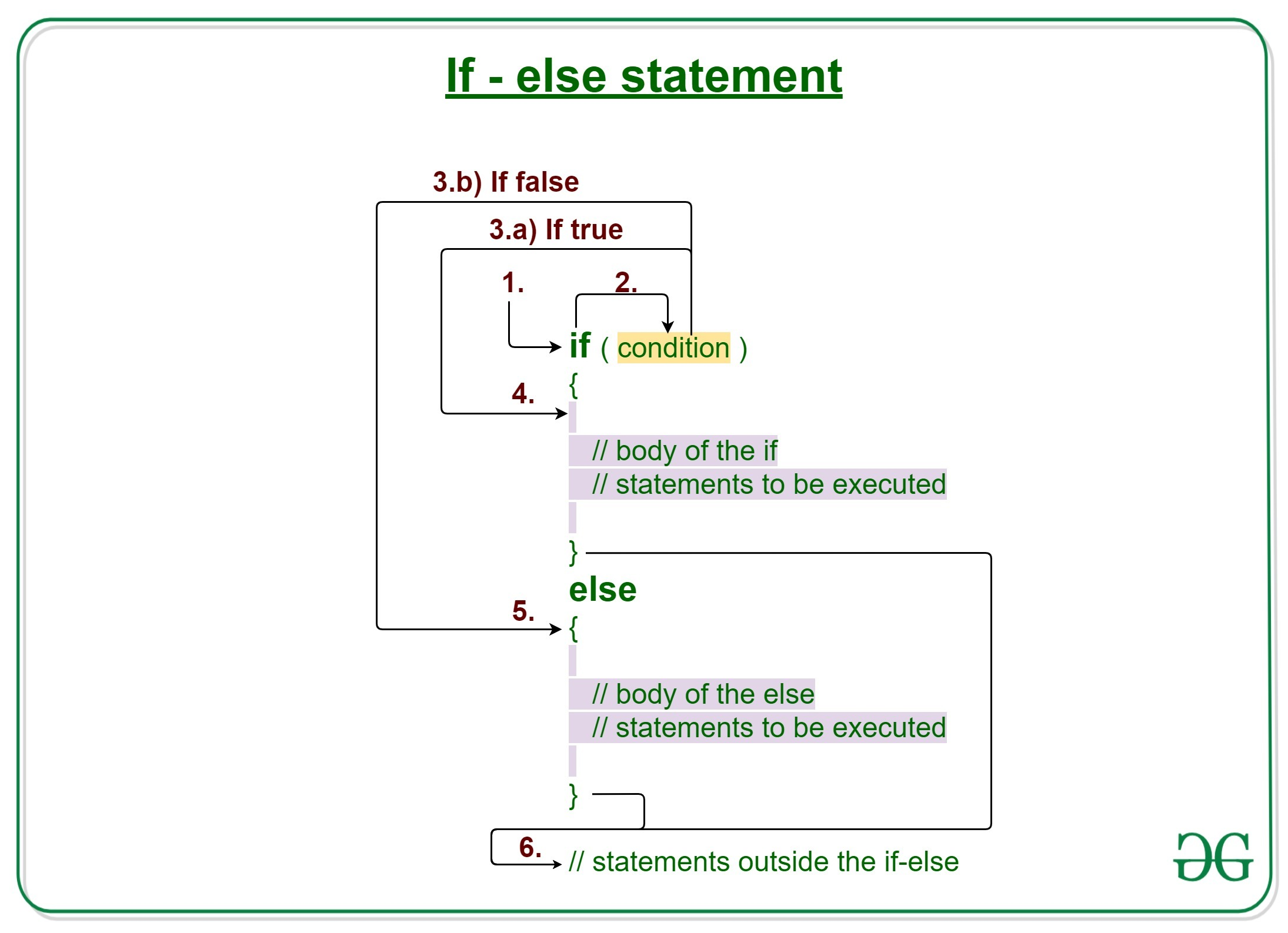# C/C++ if else statement with Examples

Decision Making in C/C++ helps to write decision driven statements and execute a particular set of code based on certain conditions.

The if statement alone tells us that if a condition is true it will execute a block of statements and if the condition is false it won’t. But what if we want to do something else if the condition is false. Here comes the C/C++ else statement. We can use the else statement with if statement to execute a block of code when the condition is false.Syntax:

```if (condition)
{
// Executes this block if
// condition is true
}
else
{
// Executes this block if
// condition is false
}```

Working of if-else statements

1. Control falls into the if block.
2. The flow jumps to Condition.
3. Condition is tested.
1. If Condition yields true, goto Step 4.
2. If Condition yields false, goto Step 5.
4. The if-block or the body inside the if is executed.
5. The else block or the body inside the else is executed.
6. Flow exits the if-else block.

Example 1:

## C

 `// C program to illustrate If statement ` ` `  `#include ` ` `  `int` `main() ` `{ ` `    ``int` `i = 20; ` ` `  `    ``// Check if i is 10 ` `    ``if` `(i == 10) ` `        ``printf``(``"i is 10"``); ` ` `  `    ``// Since is not 10 ` `    ``// Then execute the else statement ` `    ``else` `        ``printf``(``"i is 20"``); ` ` `  `    ``printf``(``"Outside if-else block"``); ` ` `  `    ``return` `0; ` `} `

## C++

 `// C++ program to illustrate if-else statement ` ` `  `#include ` `using` `namespace` `std; ` ` `  `int` `main() ` `{ ` `    ``int` `i = 20; ` ` `  `    ``// Check if i is 10 ` `    ``if` `(i == 10) ` `        ``cout << ``"i is 10"``; ` ` `  `    ``// Since is not 10 ` `    ``// Then execute the else statement ` `    ``else` `        ``cout << ``"i is 20\n"``; ` ` `  `    ``cout << ``"Outside if-else block"``; ` ` `  `    ``return` `0; ` `} `

Output:

```i is 20
Outside if-else block
```

Dry-Running Example 1:

```1. Program starts.
2. i is initialized to 20.
3. if-condition is checked. i == 10, yields false.
4. flow enters the else block.
4.a) "i is 20" is printed
5. "Outside if-else block" is printed.```

Example 2:

## C

 `// C program to illustrate If statement ` ` `  `#include ` ` `  `int` `main() ` `{ ` `    ``int` `i = 25; ` ` `  `    ``if` `(i > 15) ` `        ``printf``(``"i is greater than 15"``); ` `    ``else` `        ``printf``(``"i is smaller than 15"``); ` ` `  `    ``return` `0; ` `} `

## C++

 `// C++ program to illustrate if-else statement ` ` `  `#include ` `using` `namespace` `std; ` ` `  `int` `main() ` `{ ` `    ``int` `i = 25; ` ` `  `    ``if` `(i > 15) ` `        ``cout << ``"i is greater than 15"``; ` `    ``else` `        ``cout << ``"i is smaller than 15"``; ` ` `  `    ``return` `0; ` `} `

Output:

```i is greater than 15
```

Related Articles:

My Personal Notes arrow_drop_upCheck out this Author's contributed articles.

If you like GeeksforGeeks and would like to contribute, you can also write an article using contribute.geeksforgeeks.org or mail your article to contribute@geeksforgeeks.org. See your article appearing on the GeeksforGeeks main page and help other Geeks.

Please Improve this article if you find anything incorrect by clicking on the "Improve Article" button below.

Article Tags :
Practice Tags :

Be the First to upvote.

Please write to us at contribute@geeksforgeeks.org to report any issue with the above content.